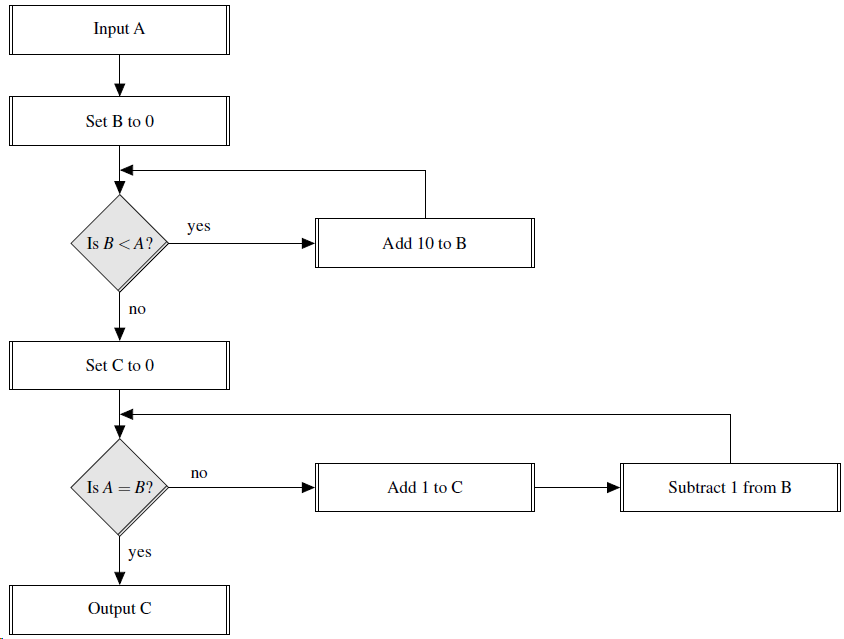Each of the values $$36$$, $$77$$, $$357$$, and $$11498$$ in turn is input to the flow diagram below.